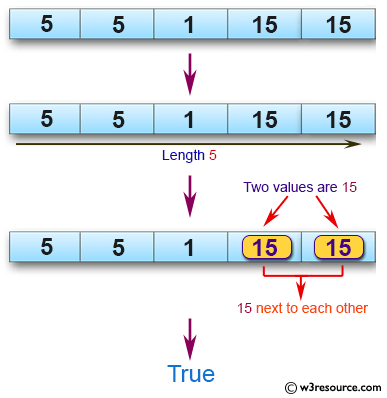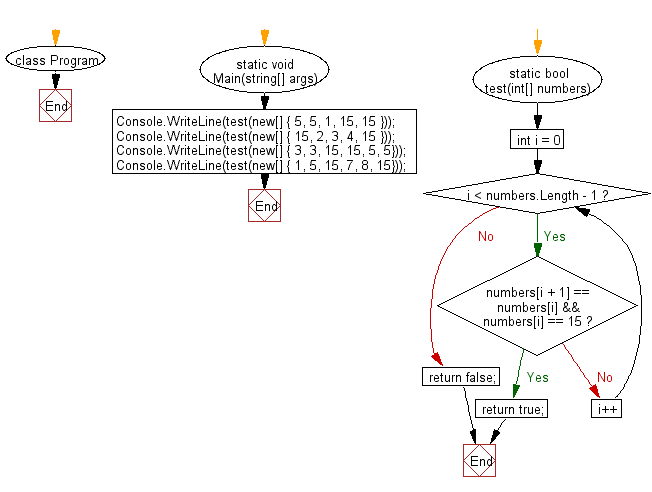﻿ C# - Find two values 15, 15 next to each other in an array# C# Sharp Basic Algorithm Exercises: Return true if there are two values 15, 15 next to each other in an array

## C# Sharp Basic Algorithm: Exercise-134 with Solution

Write a C# Sharp program to check a given array (length will be at least 2) of integers and return true if there are two values 15, 15 next to each other.

Pictorial Presentation:Sample Solution:

C# Sharp Code:

``````using System;
namespace exercises
{
class Program
{
static void Main(string[] args)
{
Console.WriteLine(test(new[] { 5, 5, 1, 15, 15 }));
Console.WriteLine(test(new[] { 15, 2, 3, 4, 15 }));
Console.WriteLine(test(new[] { 3, 3, 15, 15, 5, 5}));
Console.WriteLine(test(new[] { 1, 5, 15, 7, 8, 15}));
}
static bool test(int[] numbers)
{
for (int i = 0; i < numbers.Length - 1; i++)
{
if (numbers[i + 1] == numbers[i] && numbers[i] == 15) return true;
}
return false;
}

}
}
```
```

Sample Output:

```True
False
True
False```

Flowchart:C# Sharp Code Editor:

Improve this sample solution and post your code through Disqus

What is the difficulty level of this exercise?

Test your Programming skills with w3resource's quiz.

﻿